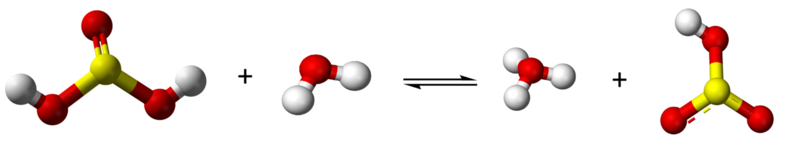14.5: Polyprotic Acids and Bases

Whereas monoprotic acids (like $$\ce{HCl}$$ pictured below) only have one hydrogen ion (aka proton - pictured below in white) to donate, polyprotic acids can donate multiple protons (see the H2SO4 molecule pictured below). Following a similar logic, polyprotic bases are bases that can accept multiple protons. With the basics of polyprotic acids and bases covered, let's dive into some of the details of how these special acids and bases work.Figure $$\PageIndex{1}$$: Lewis dot structure and ball-and-stick structure of $$\ce{HCl}$$ and $$\ce{H2SO4}$$ strong acids.

In the case of polyprotic acids and bases we can write down an equilibrium constant for each proton lost or gained. These constants are subscripted 1, 2, etc., to distinguish them. For sulfurous acid, a diprotic acid, we can, for example, write

Step 1

$\ce { H2SO3 + H2O \rightleftharpoons H3O^{+} + HSO3^{-}}$with appropriate acid constant

$K_{a\text{1}}=\frac{\text{ }[\text{ H}_{\text{3}}\text{O}^{\text{+}}\text{ }]\text{ }[\text{ HSO}_{\text{3}}^{-}\text{ }]\text{ }}{\text{ }[\text{ H}_{\text{2}}\text{SO}_{\text{3}}\text{ }]\text{ }}=\text{1}\text{.7 }\times \text{ 10}^{-\text{2}}\text{ mol L}^{-\text{1}} \label{step1}$

and

Step 2

$\text{H}\text{SO}_{3}^{-} + \text{H}_{2}\text{O} \rightleftharpoons \text{H}_{3}\text{O}^{+} + \text{SO}_{3}^{2-}$with appropriate acid constant

$K_{a\text{2}}=\frac{\text{ }[\text{ H}_{\text{3}}\text{O}^{\text{+}}\text{ }]\text{ }[\text{ SO}_{\text{3}}^{\text{2}-}\text{ }]\text{ }}{\text{ }[\text{ HSO}_{\text{3}}\text{ }]\text{ }}=\text{5}\text{.6 }\times \text{ 10}^{-\text{8}}\text{ mol L}^{-\text{1}} \label{step2}$

The carbonate ion, $$\ce{CO3^{2–}}$$, is an example of a diprotic base.

Step 1

$\text{CO}_{3}^{2-} + \text{H}_{2}\text{O} \rightleftharpoons \text{HCO}_{3}^{-} + \text{OH}^{-}$with appropriate base constant

$K_{b\text{1}}=\frac{\text{ }[\text{ HCO}_{\text{3}}^{-}\text{ }]\text{ }[\text{ OH}^{-}\text{ }]\text{ }}{\text{ }[\text{ CO}_{\text{3}}^{\text{2}-}\text{ }]\text{ }}=\text{2}\text{.1 }\times \text{ 10}^{-\text{4}}\text{ mol L}^{-\text{1}} \label{step1C}$

Step 2

$\text{HCO}_{3}^{-} + \text{H}_{2}\text{O} \rightleftharpoons \text{H}_{2}\text{CO}_{3}^{} + \text{OH}^{-}$with appropriate base constant

$K_{b\text{2}}=\frac{\text{ }[\text{ H}_{\text{2}}\text{CO}_{\text{3}}\text{ }]\text{ }[\text{ OH}^{-}\text{ }]\text{ }}{\text{ }[\text{ CO}_{\text{3}}^{-}\text{ }]\text{ }}=\text{2}\text{.4 }\times \text{ 10}^{-\text{8}}\text{ mol L}^{-\text{1}} \label{step2C}$

A general treatment of the pH of solutions of polyprotic species is beyond our intended scope, but it is worth noting that in many cases we can treat polyprotic species as monoprotic. In the case of \ce{H2SO3}\), for example, $$K_{a1} \gg K_{a2}$$ indicating that $$\ce{H2SO3}$$ is a very much stronger acid than $$\ce{HSO3^{–}}$$. This means that when $$\ce{H2SO3}$$ is dissolved in water, we can treat it as a monoprotic acid and ignore the possible loss of a second proton. Solutions of salts containing the carbonate ion, such as $$\ce{Na2CO3}$$ or $$\ce{K2CO3}$$ can be treated similarly.

Example $$\PageIndex{1}$$: pH Calculation

Find the pH of a 0.100-M solution of sodium carbonate, $$\ce{Na2CO3}$$. Use the base constant $$K_{b1} = 2.10 \times 10^{–4}\, mol\, L^{–1}$$ (Equation \ref{step1C}).

Solution

We ignore the acceptance of a second proton and treat the carbonate ion as a monoprotic base. We then have

\begin{align}\text{ }[\text{ OH}^{-}\text{ }]\text{ }=\sqrt{K_{b}c_{b}}=\sqrt{\text{2}\text{.10 }\times \text{ 10}^{-\text{4}}\text{ mol L}^{-\text{1}}\text{ }\times \text{ 0}\text{.100 mol L}^{-\text{1}}} \\\text{ }=\text{4}\text{.58 }\times \text{ 10}^{-\text{3}}\text{ mol L}^{-\text{1}} \\\end{align}

Checking, we find that

$\frac{\text{ }[\text{ OH}^{-}\text{ }]\text{ }}{c_{b}}=\frac{\text{4}\text{0.58 }\times\text{ 10}^{-\text{3}}}{\text{0}\text{.1}}\approx \text{4}\text{.6 percent}$

so that our approximation is only just valid.

We now find

$\text{pOH}=-\text{log}\left(\text{4.58}\times\text{10}^{-3}\right)= \text{2.34}$

while

$\text{pH}={14}-\text{pOH}={14}-{2.34}={11.6}$

Since the carbonate ion is a somewhat stronger base than NH3, we expect a 0.1-M solution to be somewhat more basic, as actually found.

A glance at the Ka and and Kb tables (Tables E1 and E2) reveals that most acid and base constants involve numbers having negative powers of 10. As in the case of [H3O+] and [OH], then, it is convenient to define

$\text{p}K_{a}=-\text{log}\frac{K_{a}}{\text{mol L}^{-\text{1}}}$]

${p}K_{b}=-\text{log}\frac{K_{b}}{\text{mol L}^{-\text{1}}}$

Using these definitions, the larger Ka or Kb is (i.e., the stronger an acid or base, respectively), the smaller pKaor pKb will be. For a strong acid like HNO3, Ka = 20 mol L–1 and

$\text{p}K_{a} = -\text{log}\text{ 20}=-\left(\text{1.30}\right)=-\text{1.30}$

Thus for very strong acids or bases pK values can even be negative.